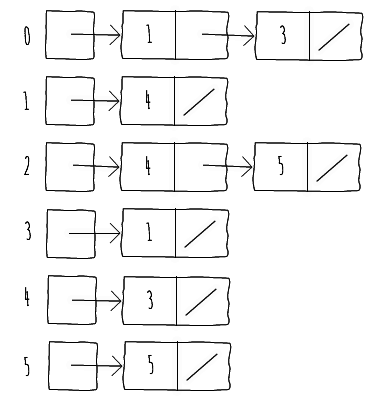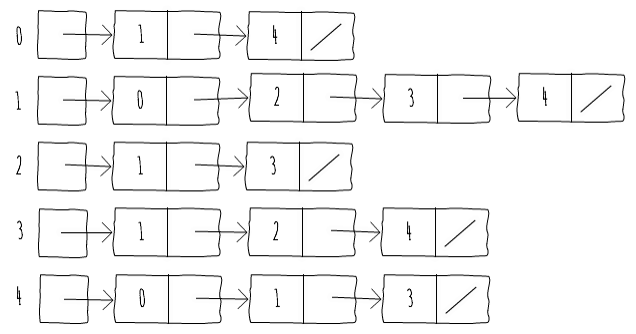•#### Beginning Java Data Structures and Algorithms#### Overview of this book

Learning about data structures and algorithms gives you a better insight on how to solve common programming problems. Most of the problems faced everyday by programmers have been solved, tried, and tested. By knowing how these solutions work, you can ensure that you choose the right tool when you face these problems. This book teaches you tools that you can use to build efficient applications. It starts with an introduction to algorithms and big O notation, later explains bubble, merge, quicksort, and other popular programming patterns. You’ll also learn about data structures such as binary trees, hash tables, and graphs. The book progresses to advanced concepts, such as algorithm design paradigms and graph theory. By the end of the book, you will know how to correctly implement common algorithms and data structures within your applications.
Title PagePackt UpsellContributorsPrefaceFree Chapter
Algorithms and ComplexitiesSorting Algorithms and Fundamental Data StructuresHash Tables and Binary Search TreesString Matching AlgorithmsGraphs, Prime Numbers, and Complexity ClassesOther Books You May EnjoyIndex## Representing Graphs

There are usually two standard ways to represent a graphG = (V, E)in a computer program:

• As a collection of adjacency lists

You can use either way to represent both directed and undirected graphs. We'll start by looking at the adjacency list representation.

The adjacency list representation of a graph consists of an array of|V|lists, one for each vertex inV. For each vertexuinV, there's a list containing all verticesv so that there is an edge connectinguandvinE.Figure 6.3shows the adjacency list representation of the directed graph inFigure 6.1:Figure 6.3: Adjacency list representation of the directed graph in Figure 6.1

For undirected graphs, we follow a similar strategy and build the adjacency list as if it were a directed graph with two edges between each pair of verticesuandv, which are(u, v)and(v, u).

Figure 6.4shows the adjacency list representation of the undirected graph inFigure 6.2: